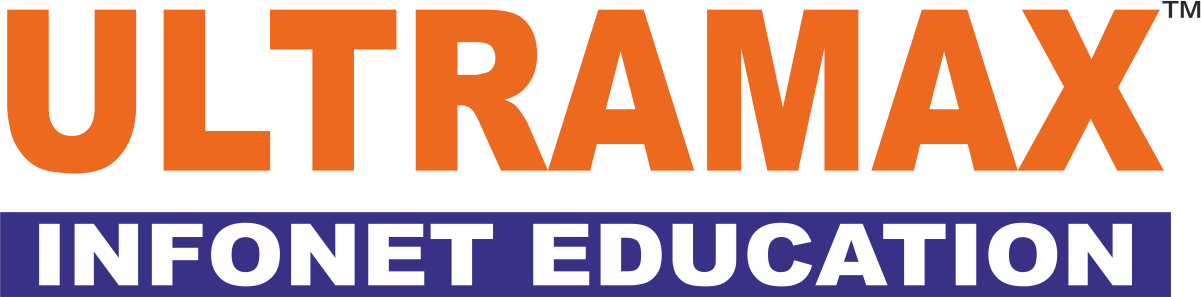Ultramax Infonet Education### Call Us

+91-9284425283 / +917498212715

### Mail Us

sales@ultramaxit.com

• # R Programming

## Statistics Theory

1.Introduction to Statistical Concepts
2.Variables and Data Types
3. Bar, Line Chart, Histogram, pie chart, Box plot
4. Measures of data-Measure of center – Mean, Median, Mode
5.Measure of Spread – Range, variance, standard deviation
6.Measure of shape – Skewness, Kurtosis
7. Statistical Distributions , probability
8. Tests
9.Test of Association – Correlation, Regression
10. Test of Inference – Chi-Square, t-test, Analysis of Variance
11. Testing of Hypothesis
12. Two-Sample t-Tests
13.One-Way ANOVA
14. ANOVA with Data from a Randomized Block Design
15. Stepwise Regression and Diagnostic tests for regression
16. Categorical Data Analysis
17. Regression Modeling

## Audience Pre-requisite

• Any graduates/ Post Graduates looking to build a career in analytics and data science
• IT professionals looking for a career switch into data science and analytics
• Professionals working in data and business analytics

## Introduction to R and R studio

1.Introduction to R and R studio
2.R Installation – R GUI and R studio, R Studio tour
3.R packages overview and understanding in-built functions – Creating/saving R script, Installing
4.Vectors – Creating numerical, logical and character vectors, Understanding size of vectors, Vector
operations, Extracting elements from vectors, Understanding different R functions like mean, sqrtetc
in the context of numerical vectors, Knowing the differences between numeric and character vector,
changing a numerical vector to factor if needed, Creating tables from vector.
5.Matrices, Data frames and Data import-Building matrices and understanding dimensions,
Extracting elements from matrices, Combining matrices by rows and by columns, Matrix operations,
Understanding Data frames and working with built in data frames, R built in data sets.
6.Visual Analytics – Bar Chart and pie chart, Histogram, scatterplot, boxplot, matrix plot, time series
plot, Understanding various packages for data visualization
7.Summarizing the data and probability distribution of data – Finding measures of center, Finding
measures of spread, Normal probability distribution
8.Testing of hypothesis and Confidence Interval – Parametric tests, Non-parametric tests, Inferences
using Confidence Interval
9.Linear Regression – Simple and Multiple Linear Regression, Stepwise Regression, Diagnostic
Tests
10.Logistic Regression – Simple and Multiple Logistic regression, Logistic regression with predictor
variables
11.Decision Trees/CART – Classification and Regression Trees Explanation
12.Confidence Interval and Sample size determination
13.Supervised and Unsupervised learning
14.Difference between classification and regression algorithms
15.Naïve Bayes Classifier
16.Principal Component Analysis
17.Factor Analysis
18.Discriminant Analysis
19.Time Series Analysis
20.k-means clustering

Don't Miss Out on Our Business Resources